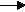gcsescience.com                                       11                                       gcsescience.com

Moles

Calculating the Mass of a Product from a Reaction.
Basic Method.

Example 2 (see example 1).
What mass of sodium oxide will be formed
by burning 20 g  of sodium in air?

1) Find how many moles of sodium are present in 20 g  of sodium.

The RAM of sodium is 23.

moles = mass ÷ RAM

moles = 20 ÷ 23
= 0·869 moles of sodium.

2) Write the equation for the reaction.

sodium + oxygensodium oxide.
4Na(s)  +    O2(g)2Na
2O(s)

Use the big numbers to find the proportion of reactant to product.
4Na makes 2Na
2O, so 2Na makes 1Na2O,
so the proportion is 2 to 1.
0·869 moles of sodium will make (0·869 ÷ 2) moles
of sodium oxide,
= 0·435 moles of sodium oxide.

3) Find the mass of 0·435 moles of sodium oxide.

RFM of Na2O is (2 x 23) + (1 x 16)  =  62.

mass = moles x RFM

mass = 0·435 x 62
= 26·97 g  of sodium oxide.

So, burning 20 g  of sodium in air will make
26·97 g  of sodium oxide.

See the next page for the advanced method.

gcsescience.com       The Periodic Table       Index       Moles Quiz       gcsescience.com